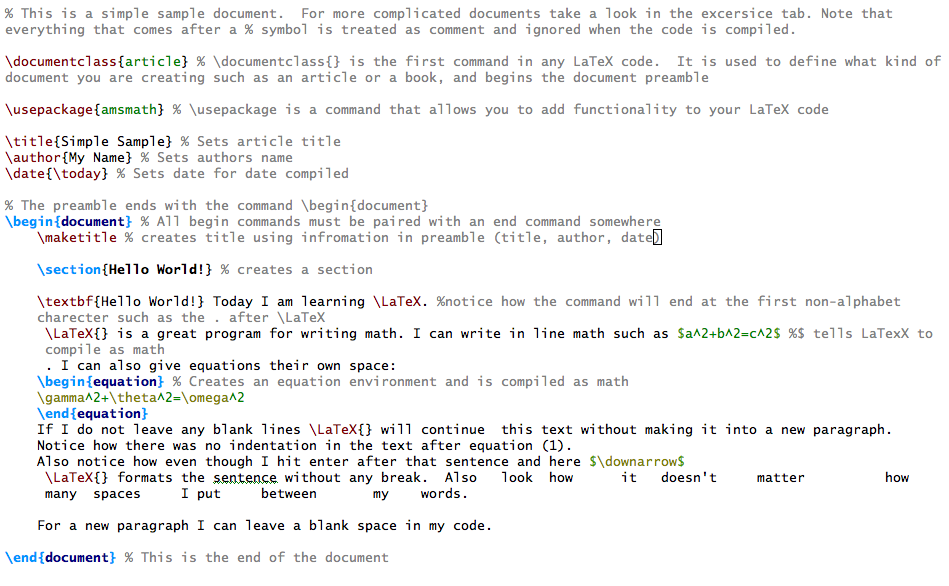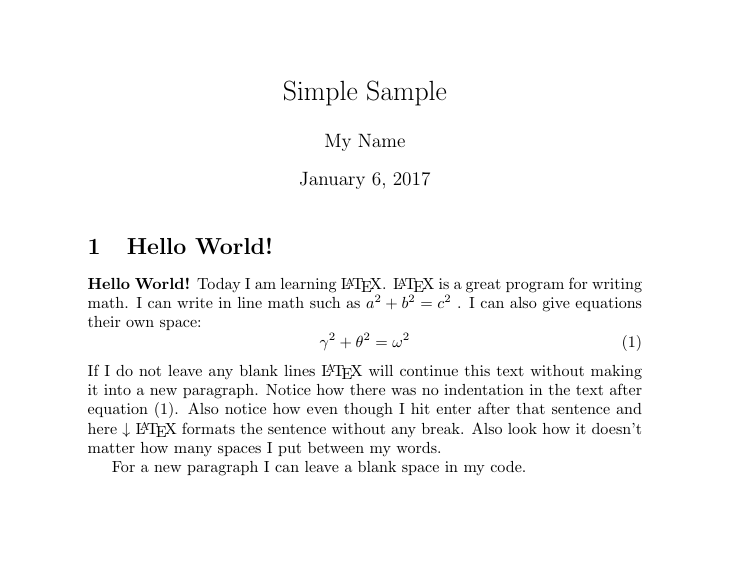# Getting Started with LaTeX

LaTeX is a typesetting program useful for mathematical and scientific writings or publications. This guide provides an overview of how to get started with LaTeX, as well as resources and exercise to help new users of the program.

## Introduction

Below is the LaTeX code and the PDF output for a simple sample document.  First you will see a readable version of the code followed by the LaTeX PDF output.  At the bottom of the you will find a easy to copy version of the code if you would like to play around with and compile it on your computer.## Compiled code## Copyable text

% This is a simple sample document.  For more complicated documents take a look in the exercise tab. Note that everything that comes after a % symbol is treated as comment and ignored when the code is compiled.

\documentclass{article} % \documentclass{} is the first command in any LaTeX code.  It is used to define what kind of document you are creating such as an article or a book, and begins the document preamble

\usepackage{amsmath} % \usepackage is a command that allows you to add functionality to your LaTeX code

\title{Simple Sample} % Sets article title
\author{My Name} % Sets authors name
\date{\today} % Sets date for date compiled

% The preamble ends with the command \begin{document}
\begin{document} % All begin commands must be paired with an end command somewhere
\maketitle % creates title using information in preamble (title, author, date)

\section{Hello World!} % creates a section

\textbf{Hello World!} Today I am learning \LaTeX. %notice how the command will end at the first non-alphabet charecter such as the . after \LaTeX
\LaTeX{} is a great program for writing math. I can write in line math such as $a^2+b^2=c^2$ %$tells LaTexX to compile as math . I can also give equations their own space: \begin{equation} % Creates an equation environment and is compiled as math \gamma^2+\theta^2=\omega^2 \end{equation} If I do not leave any blank lines \LaTeX{} will continue this text without making it into a new paragraph. Notice how there was no indentation in the text after equation (1). Also notice how even though I hit enter after that sentence and here$\downarrow\$
\LaTeX{} formats the sentence without any break.  Also   look  how      it   doesn't     matter          how    many  spaces     I put     between       my    words.

For a new paragraph I can leave a blank space in my code.

\end{document} % This is the end of the document